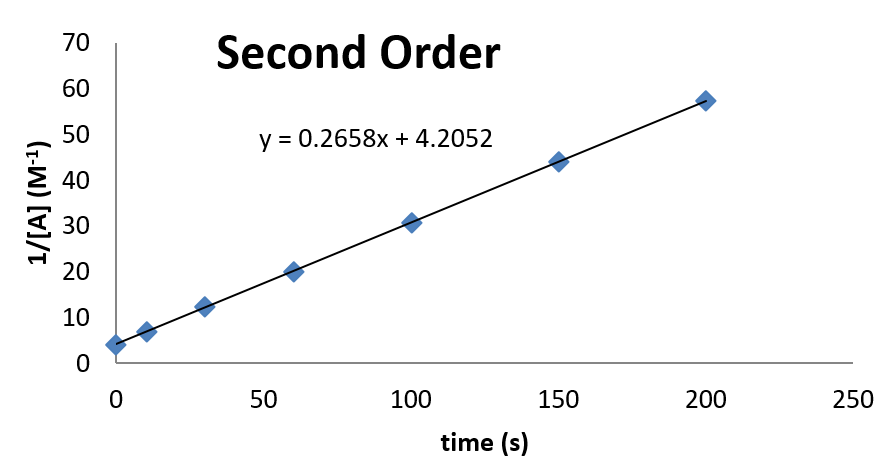# 11.6: 2nd order Rate Laws

$$\newcommand{\vecs}{\overset { \rightharpoonup} {\mathbf{#1}} }$$ $$\newcommand{\vecd}{\overset{-\!-\!\rightharpoonup}{\vphantom{a}\smash {#1}}}$$$$\newcommand{\id}{\mathrm{id}}$$ $$\newcommand{\Span}{\mathrm{span}}$$ $$\newcommand{\kernel}{\mathrm{null}\,}$$ $$\newcommand{\range}{\mathrm{range}\,}$$ $$\newcommand{\RealPart}{\mathrm{Re}}$$ $$\newcommand{\ImaginaryPart}{\mathrm{Im}}$$ $$\newcommand{\Argument}{\mathrm{Arg}}$$ $$\newcommand{\norm}{\| #1 \|}$$ $$\newcommand{\inner}{\langle #1, #2 \rangle}$$ $$\newcommand{\Span}{\mathrm{span}}$$ $$\newcommand{\id}{\mathrm{id}}$$ $$\newcommand{\Span}{\mathrm{span}}$$ $$\newcommand{\kernel}{\mathrm{null}\,}$$ $$\newcommand{\range}{\mathrm{range}\,}$$ $$\newcommand{\RealPart}{\mathrm{Re}}$$ $$\newcommand{\ImaginaryPart}{\mathrm{Im}}$$ $$\newcommand{\Argument}{\mathrm{Arg}}$$ $$\newcommand{\norm}{\| #1 \|}$$ $$\newcommand{\inner}{\langle #1, #2 \rangle}$$ $$\newcommand{\Span}{\mathrm{span}}$$$$\newcommand{\AA}{\unicode[.8,0]{x212B}}$$

If the reaction follows a second order rate law, the some methodology can be employed. The rate can be written as

$-\dfrac{d[A]}{dt} = k [A]^2 \label{eq1A}$

The separation of concentration and time terms (this time keeping the negative sign on the left for convenience) yields

$-\dfrac{d[A]}{[A]^2} = k \,dt \nonumber$

The integration then becomes

$- \int_{[A]_o}^{[A]} \dfrac{d[A]}{[A]^2} = \int_{t=0}^{t}k \,dt \label{eq1}$

And noting that

$- \dfrac{dx}{x^2} = d \left(\dfrac{1}{x} \right) \nonumber$

the result of integration Equation \ref{eq1} is

$\dfrac{1}{[A]} -\dfrac{1}{[A]_o} = kt \nonumber$

or

$\dfrac{1}{[A]} = \dfrac{1}{[A]_o} + kt \nonumber$

And so a plot of $$1/[A]$$ as a function of time should produce a linear plot, the slope of which is $$k$$, and the intercept of which is $$1/[A]_0$$.

Other 2nd order rate laws are a little bit trickier to integrate, as the integration depends on the actual stoichiometry of the reaction being investigated. For example, for a reaction of the type

$A + B \rightarrow P \nonumber$

That has rate laws given by

$-\dfrac{d[A]}{dt} = k [A][B] \nonumber$

and

$-\dfrac{d[B]}{dt} = k [A][B] \nonumber$

the integration will depend on the decrease of [A] and [B] (which will be related by the stoichiometry) which can be expressed in terms the concentration of the product [P].

$[A] = [A]_o – [P] \label{eqr1}$

and

$[B] = [B]_o – [P]\label{eqr2}$

The concentration dependence on $$A$$ and $$B$$ can then be eliminated if the rate law is expressed in terms of the production of the product.

$\dfrac{d[P]}{dt} = k [A][B] \label{rate2}$

Substituting the relationships for $$[A]$$ and $$[B]$$ (Equations \ref{eqr1} and \ref{eqr2}) into the rate law expression (Equation \ref{rate2}) yields

$\dfrac{d[P]}{dt} = k ( [A]_o – [P]) ([B] = [B]_o – [P]) \label{rate3}$

Separation of concentration and time variables results in

$\dfrac{d[P]}{( [A]_o – [P]) ([B] = [B]_o – [P])} = k\,dt \nonumber$

Noting that at time $$t = 0$$, $$[P] = 0$$, the integrated form of the rate law can be generated by solving the integral

$\int_{[A]_o}^{[A]} \dfrac{d[P]}{( [A]_o – [P]) ([B]_o – [P])} = \int_{t=0}^{t} k\,dt \nonumber$

Consulting a table of integrals reveals that for $$a \neq b$$,

$\int \dfrac{dx}{(a-x)(b-x)} = \dfrac{1}{b-a} \ln \left(\dfrac{b-x}{a-x} \right) \nonumber$

Applying the definite integral (as long as $$[A]_0 \neq [B]_0$$) results in

$\left. \dfrac{1}{[B]_0-[A]_0} \ln \left( \dfrac{[B]_0-[P]}{[A]_0-[P]} \right) \right |_0^{[A]} = \left. k\, t \right|_0^t \nonumber$

$\dfrac{1}{[B]_0-[A]_0} \ln \left( \dfrac{[B]_0-[P]}{[A]_0-[P]} \right) -\dfrac{1}{[B]_0-[A]_0} \ln \left( \dfrac{[B]_0}{[A]_0} \right) =k\, t \label{finalint}$

Substituting Equations \ref{eqr1} and \ref{eqr2} into Equation \ref{finalint} and simplifying (combining the natural logarithm terms) yields

$\dfrac{1}{[B]_0-[A]_0} \ln \left( \dfrac{[B][A]_o}{[A][B]_o} \right) = kt \nonumber$

For this rate law, a plot of $$\ln([B]/[A])$$ as a function of time will produce a straight line, the slope of which is

$m = ([B]_0 – [A]_0)k. \nonumber$

In the limit at $$[A]_0 = [B]_0$$, then $$[A] = [B]$$ at all times, due to the stoichiometry of the reaction. As such, the rate law becomes

$\text{rate} = k [A]^2 \nonumber$

and integrate direct like in Equation \ref{eq1A} and the integrated rate law is (as before)

$\dfrac{1}{[A]} = \dfrac{1}{[A]_o} + kt \nonumber$

##### Example $$\PageIndex{2}$$: Confirming Second Order Kinetics

Consider the following kinetic data. Use a graph to demonstrate that the data are consistent with second order kinetics. Also, if the data are second order, determine the value of the rate constant for the reaction.

 time (s) [A] (M) 0 10 30 60 100 150 200 0.238 0.161 0.098 0.062 0.041 0.029 0.023
###### Solution

The plot looks as follows:From this plot, it can be seen that the rate constant is 0.2658 M-1 s-1. The concentration at time $$t = 0$$ can also be inferred from the intercept.

 This integral form can be generated by using the method of partial fractions. See (House, 2007) for a full derivation.

This page titled 11.6: 2nd order Rate Laws is shared under a CC BY-NC-SA 4.0 license and was authored, remixed, and/or curated by Patrick Fleming.